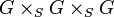Group scheme

(diff) ← Older revision | Latest revision (diff) | Newer revision → (diff)
This is a variation of group|Find other variations of group | Read a survey article on varying group

Definition

Abstract definition

A group scheme over$S$ is a group object in a category of schemes with fiber products and final object$S$.

Concrete definition

A group scheme over$S$ is a$S$-scheme$G$ (in a category of schemes that admits fiber products over$S$) equipped with the following operations:

Operation name Arity of operation Operation description and notation
Multiplication or product 2 A binary operation$\mu: G \times_S G \to G$
Identity element (or neutral element) 0 A map$e:S \to G$.
Inverse map 1 A unary operation$\iota: G \to G$.

satisfying the following compatibility conditions:

Condition name Arity of space from which the maps are equal Condition description Comments
Associativity 3 We have that$\mu \circ (\mu \times_S \operatorname{id}) = \mu \circ (\operatorname{id} \times_S \mu)$ as maps from$G \times_S G \times_S G$ Note: We are abusing notation somewhat and treating$G \times_S G \times_S G$ as well defined, but the rigorous way of doing it is to actually use the associativity isomorphism to go between the two versions of it.
Identity element (or neutral element) 1 We have$\operatorname{id} = \mu \circ (e \times_S \operatorname{id}) = \mu \circ \mu \circ (\operatorname{id} \times_S e)$ as maps from$G$ to$G$. Note that we are using the canonical identification of$G$ with$G \times_S S$ and$S \times_S G$.
Inverse element 1 We have$\mu \circ (\operatorname{id} \times_S \iota) = \mu \circ (\iota \times_S \operatorname{id}) = e$ as maps from$G$ to$G$. Note that the map$e$ is a map$S \to G$, but can be treated as a map$G \to G$ via pre-composition with the unique map from$G$ to$S$, on account of$S$ being a final object.